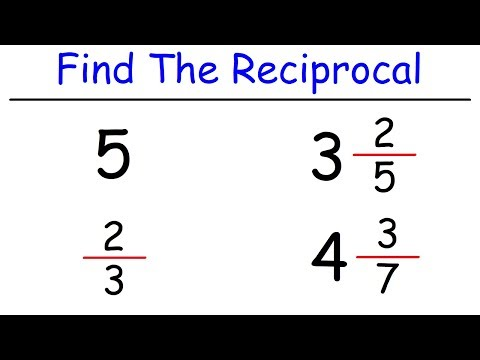# Calculatate the reciprocal or multiplicative inverse of 2 4/12

Use our calculator to get the reciprocal of 2 4/12. Also, learn what is the reciprocal of a number or of a fraction as well as use our step-by-step calculator.

### Reciprocal of a Number or of a Fraction Calculator

Ex.: 2, 2.33, 1/2, 2 1/2, etc.
Note that 2 1/2 means two plus a half = 2 + 1/2

### Step-by-Step Solution:

...

Quote of the day...

## What Does a Multiplicative Inverse or Reciprocal Mean?

A multiplicative inverse or reciprocal for a number n, denoted by 1n or n−1 (n to the power of minus one), is a number which when multiplied by n, their product is 1. In other words, the reciprocal of any number is one divided by that number. The reciprocal of a fraction xy is yx. To find the multiplicative inverse of a real number, just divide 1 by that number. Examples:

• The reciprocal of 7 is one senventh = 1/7 = 0,142857142857...)
• The reciprocal of 0.333... is 1 divided by 0.333..., or 3.

## Video on How To Find The Reciprocal of Whole Numbers, Fractions and Mixed Numbers

This excellent video explains how to find the reciprocal of a whole number, a fraction, as well as, the reciprocal of a mixed number.↼ Click on the image to watch the video.

### Disclaimer

While every effort is made to ensure the accuracy of the information provided on this website, neither this website nor its authors are responsible for any errors or omissions. Therefore, the contents of this site are not suitable for any use involving risk to health, finances or property.